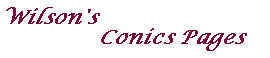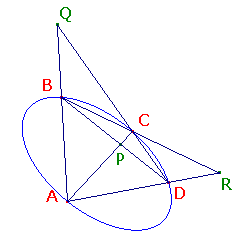If the vertices of a quadrilateral lie on a conic,
then the line joining the intersections of opposite sides
is the polar of the intersection of the lines joining opposite vertices.

This suggests a construction for the polar of a point P:
Take two lines through P, meeting the conic at A,B,C and D.
Then the polar of P is the line joining the intersections of AB,CD and of AD,BC.
Unfortunately, this will fail if P lies on the conic (when it should return the tangent).The dual of this is

If the sides of a quadrilateral touch a conic,
then the intersection of the lines joining opposite vertices
is the pole of the line joining the intersections of opposite sides.

Here, we may observe that to say that a point P is the pole of a line QR
is exactly the same as saying that QR is the polar of P.
Then we see that the two theorems assert the same property

If a quadrilateral is inscribed in, or circumscribed round a conic,
then the line joining the intersections of opposite sides is
the polar of the inersection of the diagonals.Indeed, corresponding inscribed and circumscribed quadrilaterals
have the following remarkable property.
I have chosen the name since it involves two quadrilaterals,
each of which is the dual of the other.